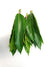Best Deals

Best Deals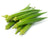Rs. 18.00
Rs. 25.00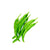Rs. 18.00
Rs. 20.00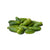Rs. 15.00
Rs. 24.00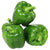Rs. 22.00
Rs. 35.00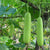Rs. 30.00
Rs. 37.50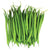Rs. 14.00
Rs. 35.00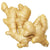Rs. 15.00
Rs. 35.00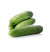Rs. 15.00
Rs. 25.00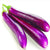Rs. 14.00
Rs. 23.50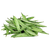Rs. 17.50
Rs. 30.00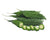Rs. 35.00
Rs. 42.00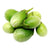Rs. 14.00
Rs. 23.50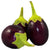Rs. 14.00
Rs. 22.50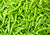Rs. 18.00
Rs. 20.00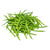Rs. 15.00
Rs. 29.50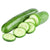Rs. 39.00
Rs. 46.50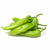Rs. 22.00
Rs. 29.75
Sold Out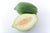Rs. 45.00
Rs. 70.00
Sold Out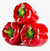Rs. 96.50
Rs. 125.00
Sold Out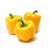Rs. 96.50
Rs. 125.00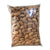Rs. 275.00
Rs. 350.00
Sold Out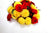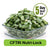Rs. 30.00
Rs. 45.00
Sold Out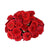Rs. 25.00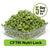Rs. 28.00
Rs. 42.00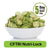Rs. 27.00
Rs. 36.25
Sold Out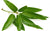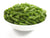Rs. 27.00
Rs. 42.00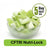Rs. 20.00
Rs. 28.00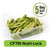Rs. 27.00
Rs. 35.25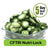Rs. 32.00
Rs. 40.00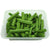Rs. 58.00
Rs. 80.00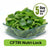Rs. 29.00
Rs. 45.00
Sold Out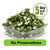Rs. 29.00
Rs. 38.00
Sold OutRs. 29.00
Rs. 38.00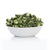Rs. 32.00
Rs. 40.00
Sold Out